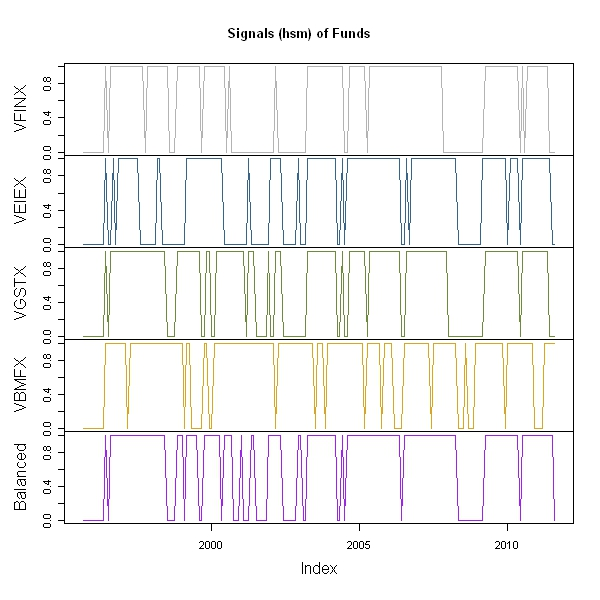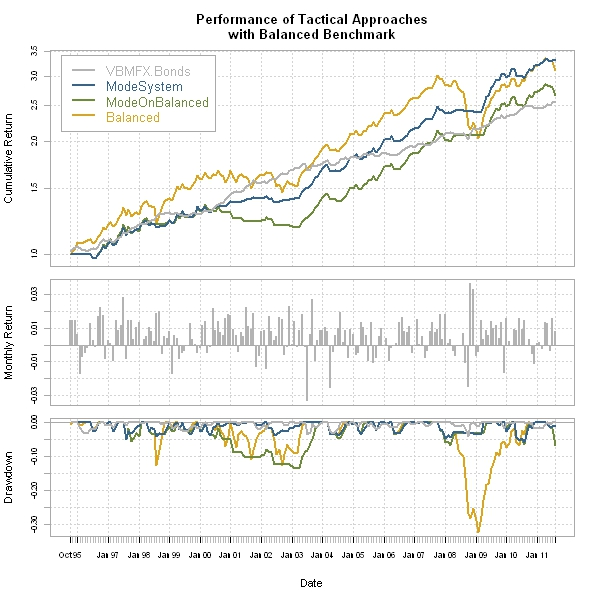Want to share your content on R-bloggers? click here if you have a blog, or here if you don't.

Let’s take Modest Modeest for Moving Average one step further and use it in a basic tactical allocation system using Vanguard funds.  THIS IS NOT INVESTMENT ADVICE AND VERY EASILY MIGHT CAUSE LARGE LOSSES.  VANGUARD FUNDS IMPOSE EARLY REDEMPTION FEES THAT RENDER THIS NOT FEASIBLE.

If we use US Equities (vfinx for S&P 500), International Developed (vgstx), and Emerging (veiex) to fill the 60% equities slot in a portfolio and then add 40% US Bonds (vbmfx), we can fairly closely resemble a typical balanced allocation.  Instead of buy and hold, let’s only buy and hold when each is above its respective 10 month mode, but sell whenever it falls below that mode.  The result is fairly attractive especially in terms of drawdown reduction.From TimelyPortfolioFrom TimelyPortfolioFrom TimelyPortfolio

Ultimately, to choose mode over mean, we should evaluate versus the Mebane Faber 10 month moving average system.  The results are far from conclusive, but both clearly beat the balanced benchmark in terms of drawdown.  Since I would think it is much easier to explain mean to clients, I would say that Mebane’s system wins in this very simple framework.  However, this is only start.  Please let me know any discoveries as you apply to a much more diverse set of allocation options.From TimelyPortfolio

`require(quantmod)require(modeest)require(PerformanceAnalytics)   start = "1990-12-31"end = Sys.Date()   #use Vanguard funds as proxy for asset classes#due to Vanguard fees for early redemption#these funds not appropriate for Tactical systemtckrs <- c("VFINX","VEIEX","VGSTX","VBMFX")getSymbols(tckrs, from=start, to=end, adjust=TRUE)for (i in 1:length(tckrs)) {	assign(tckrs[i],to.monthly(get(tckrs[i]))[,4])}   funds <- na.omit(merge(VFINX[,1],VEIEX[,1],VGSTX[,1],VBMFX[,1]))#get dates to include day so format is YYYY-MM-DDindex(funds) <- as.Date(index(funds))colnames(funds) <- tckrs   #get 1 month changefunds.roc <- ROC(funds,type="discrete",n=1)#add a balanced benchmark#20% of each equity fund for 60% total equities#40% of US bondsfunds.roc <- merge(funds.roc,	0.2*funds.roc[,1]+0.2*funds.roc[,2]+0.2*funds.roc[,3]+0.4*funds.roc[,4])funds.roc[1,5] <- 0colnames(funds.roc) <- c(colnames(funds),"Balanced")   #combine all the funds into one xtsfunds <- merge(funds,cumprod(1+funds.roc[,5]))#charts.PerformanceSummary(funds.roc,#	main="Vanguard Funds for US, Emerging, Intl Stocks and US Bonds#	with a 60/40 Balanced Allocation")   #set width to be 10 months similar to Mebane Faber 10 month moving averagewidth = 10#set up system.signal with same number of columns and rows as fundssystem.signal <- funds#loop through each column since I could not use apply with mode calculationfor (m in 1:NCOL(system.signal)) {	#another for loop to do the rolling mode since it also does not work with apply	for (n in width:NROW(system.signal[,m])) {		md <- mlv(funds[(n-width):n,m], method = "lientz", bw=0.4)		ifelse(n==width,			md.hist <- as.numeric(md),			md.hist <- rbind(md.hist,as.numeric(md)))	}	#make into nice xts	md.xts <- as.xts(as.data.frame(md.hist),		order.by=index(system.signal[(width):NROW(system.signal)]))	#put rolling modes into system.signal	system.signal[(width):NROW(system.signal),m] <- md.hist}   #jpeg(filename="mode chart.jpg",#	quality=100,width=6.25, height = 6.25,  units="in",res=96)plot.zoo(system.signal,main="Modes (lientz) of Funds",nc=1,	col=c("gray70","steelblue4","darkolivegreen4","goldenrod","purple"))#dev.off()   #difficult way to get 1 for in and 0 for out#but the ifelse gave me an index increasing order error#will use apply insteadsystem.signal <- funds > system.signalsystem.signal[,1:length(tckrs)] <- apply(system.signal[,1:length(tckrs)],MARGIN=2,FUN=as.numeric)ret.signal <- lag(system.signal,k=1) * funds.roc[,1:5]ret.compare <- merge(funds.roc[,4],	0.2*ret.signal[,1]+0.2*ret.signal[,2]+0.2*ret.signal[,3]+0.4*ret.signal[,4],	ret.signal[,5],	funds.roc[,5])colnames(ret.compare) <- c("VBMFX.Bonds","ModeSystem","ModeOnBalanced","Balanced")   #jpeg(filename="signal chart.jpg",#	quality=100,width=6.25, height = 6.25,  units="in",res=96)plot.zoo(system.signal,main="Signals (hsm) of Funds",nc=1,	col=c("gray70","steelblue4","darkolivegreen4","goldenrod","purple"))#dev.off()   #jpeg(filename="performance summary no average.jpg",#	quality=100,width=6.25, height = 6.25,  units="in",res=96)charts.PerformanceSummary(ret.compare,ylog=TRUE,	main="Performance of Tactical Approaches	with Balanced Benchmark",	colorset=c("gray70","steelblue4","darkolivegreen4","goldenrod","purple"),	cex.legend=1.2,lwd=2)#dev.off()     #now let's compare to Mebane Faber 10-month moving average strategy#will do in 1 line since there are multiple examples of how#to implement this in Rret.average <- lag(as.xts(apply(	funds > apply(funds[,1:5],MARGIN=2,FUN=runMean,10),MARGIN=2,as.numeric),order.by=index(funds)),k=1) * funds.rocret.compare <- merge(ret.compare,	0.2*ret.average[,1]+0.2*ret.average[,2]+0.2*ret.average[,3]+0.4*ret.average[,4])colnames(ret.compare) <- c(colnames(ret.compare)[1:(NCOL(ret.compare)-1)],"Faber10moAvg")   #jpeg(filename="performance summary with Faber average.jpg",#	quality=100,width=6.25, height = 6.25,  units="in",res=96)charts.PerformanceSummary(ret.compare,ylog=TRUE,	main="Performance of Tactical Approaches	with Balanced Benchmark and Mebane Faber 10mo Mov Avg",	colorset=c("gray70","steelblue4","darkolivegreen4","goldenrod","purple"),	cex.legend=1.2,lwd=2)#dev.off()`